# 【收藏】用 Pytorch 理解卷积网络

2020/04/10 20:49卷积神经网络的解释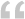比较MLPS和CNNS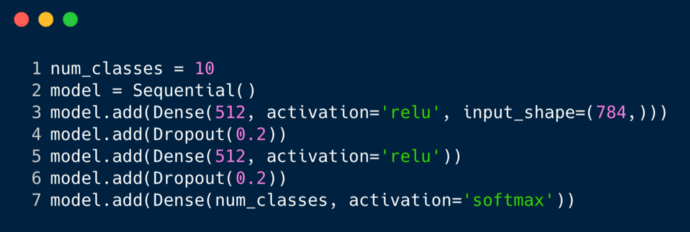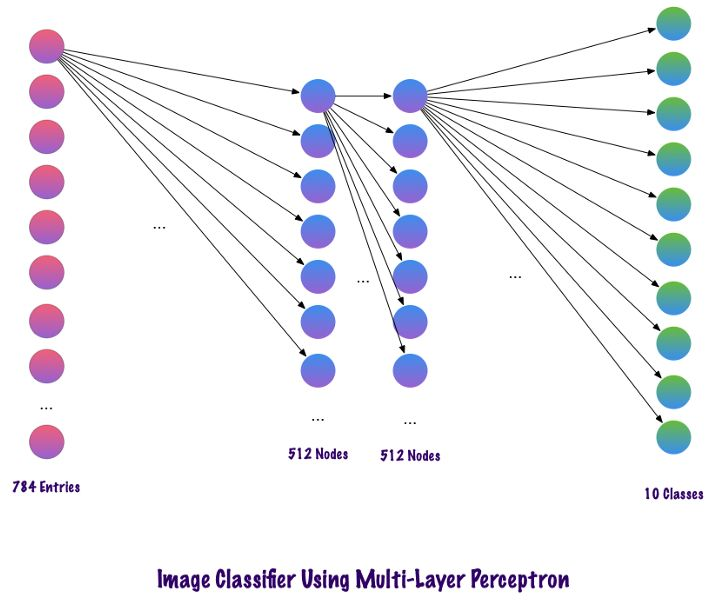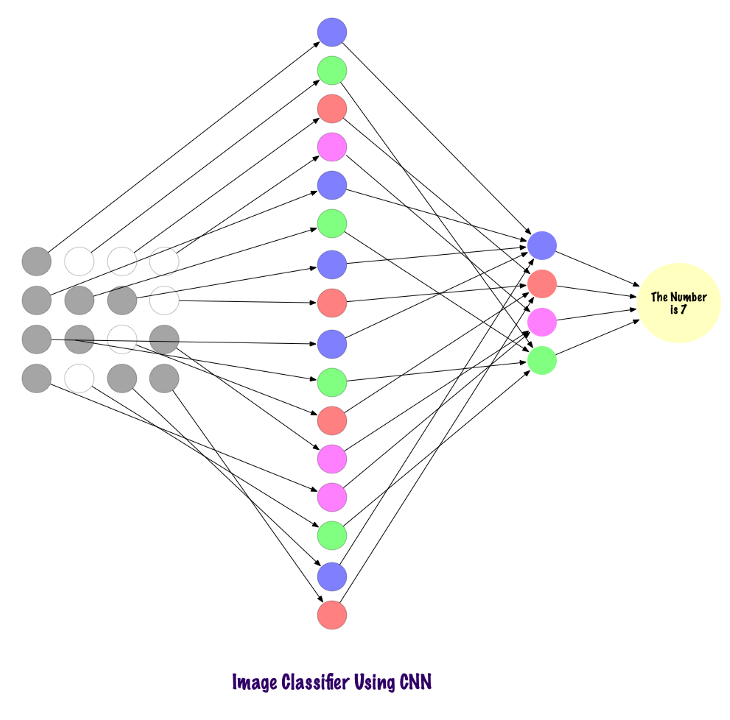计算机如何看图像？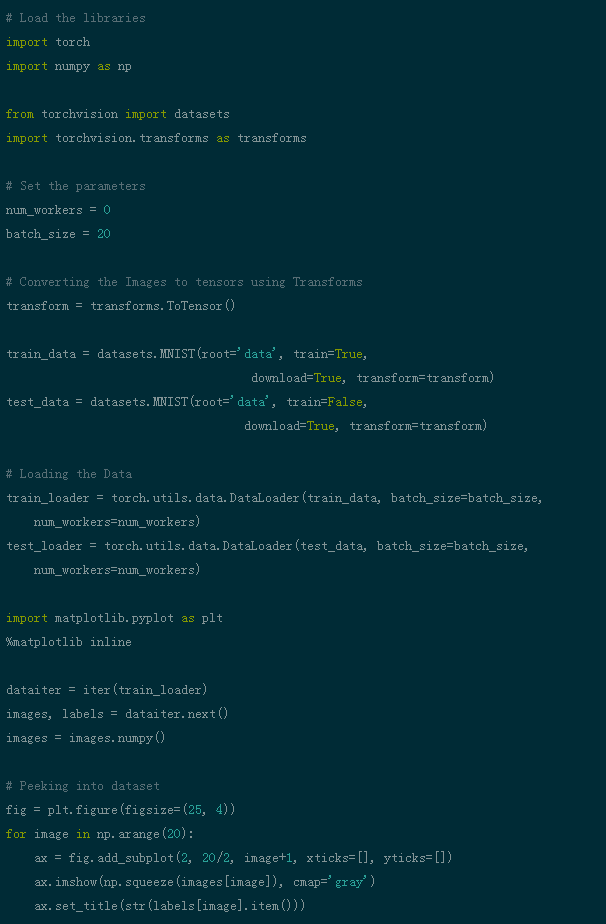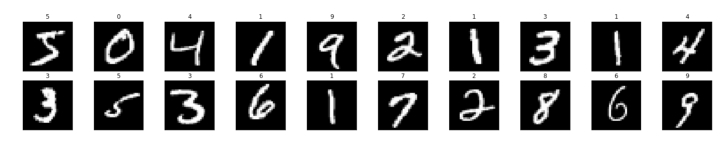（在GitHub上可找到此代码）



img = np.squeeze(images)fig = plt.figure(figsize = (12,12)) ax = fig.add_subplot(111)ax.imshow(img, cmap= gray )width, height = img.shapethresh = img.max()/2.5for x in range(width):    for y in range(height):        val = round(img[x][y],2) if img[x][y] !=0 else 0        ax.annotate(str(val), xy=(y,x),            color= white  if img[x][y]<thresh else  black )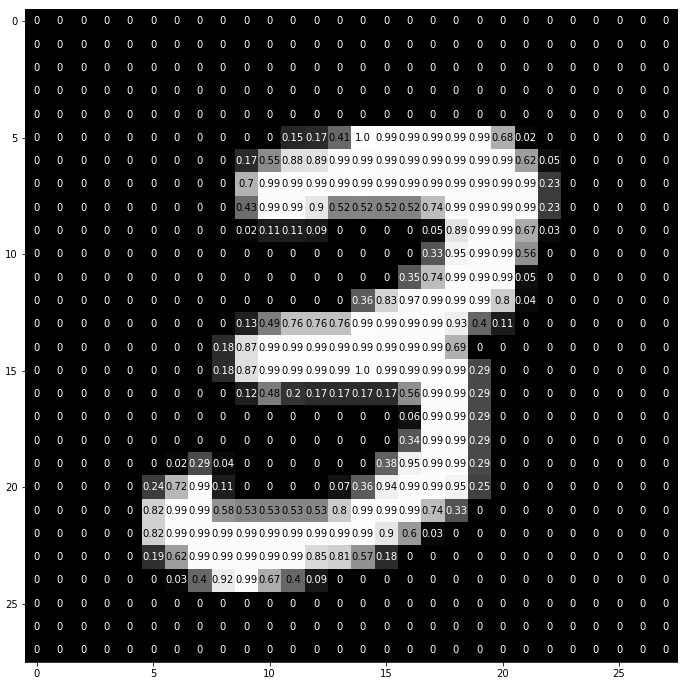建立自己的滤波器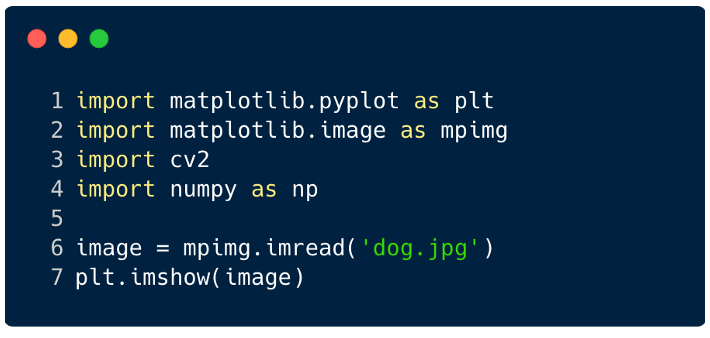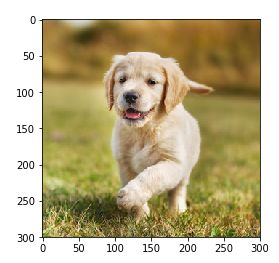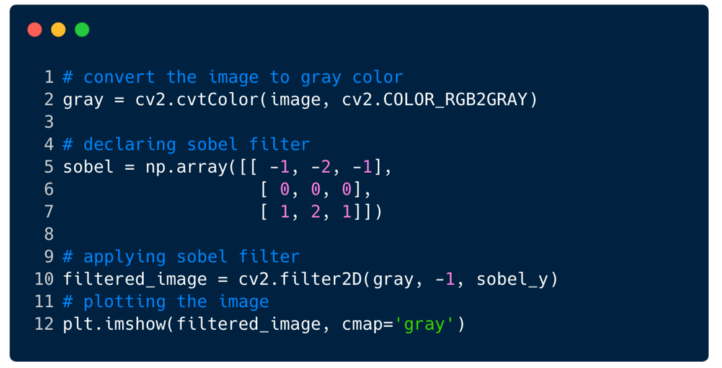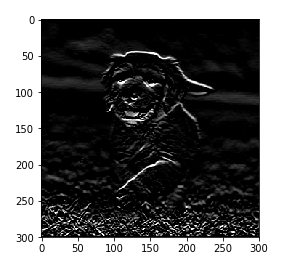## 完整的卷积神经网络（CNNS）

1.卷积层

2.池化层

3.全连接层

#### CNN各层的作用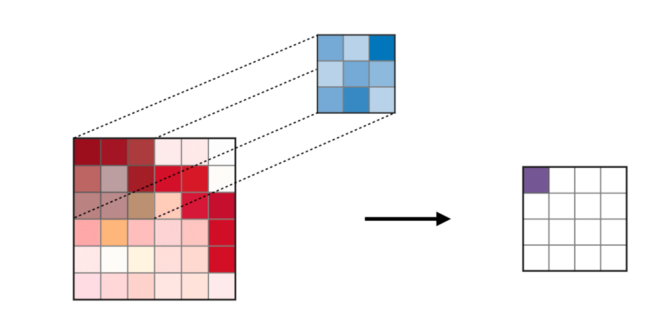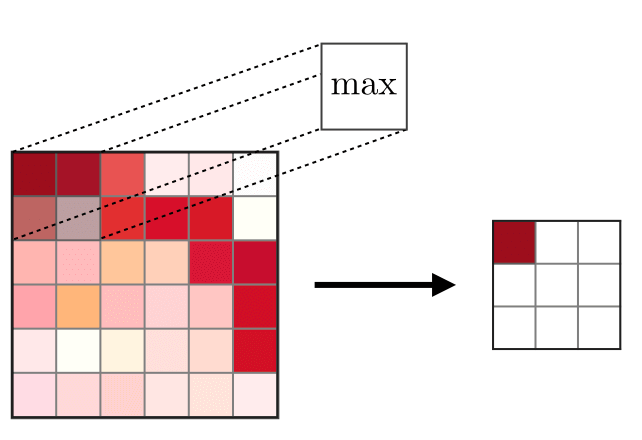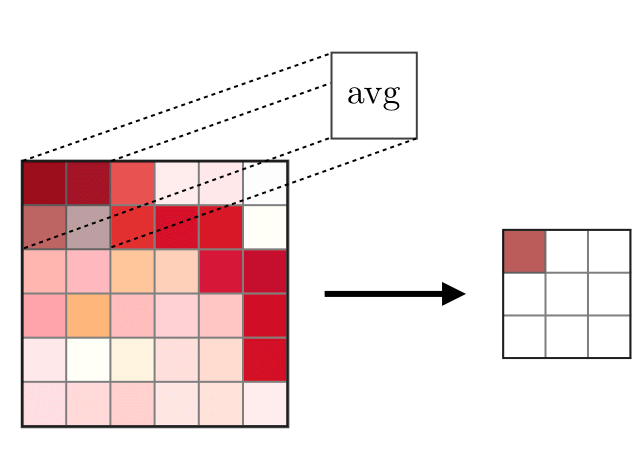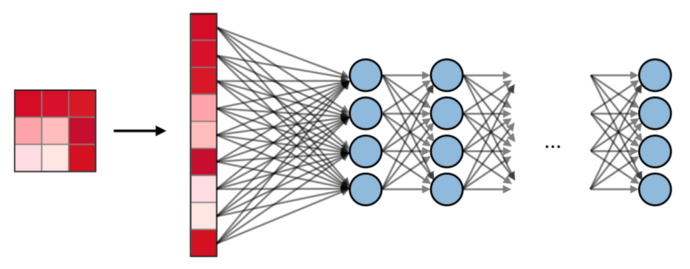在Pytorch可视化CNN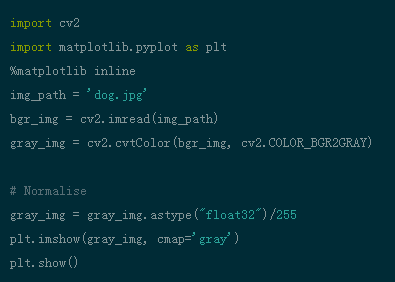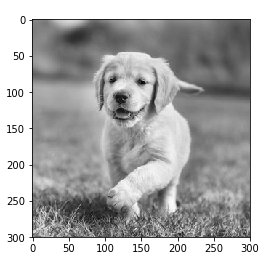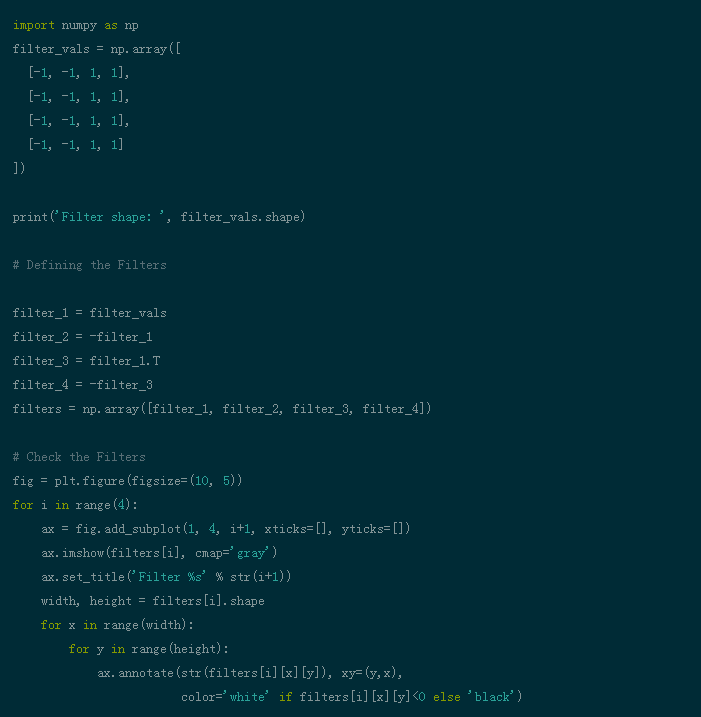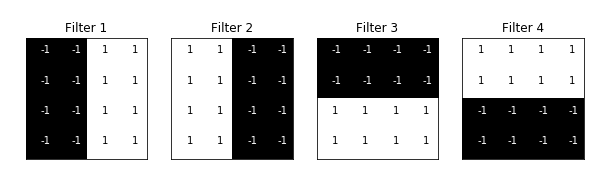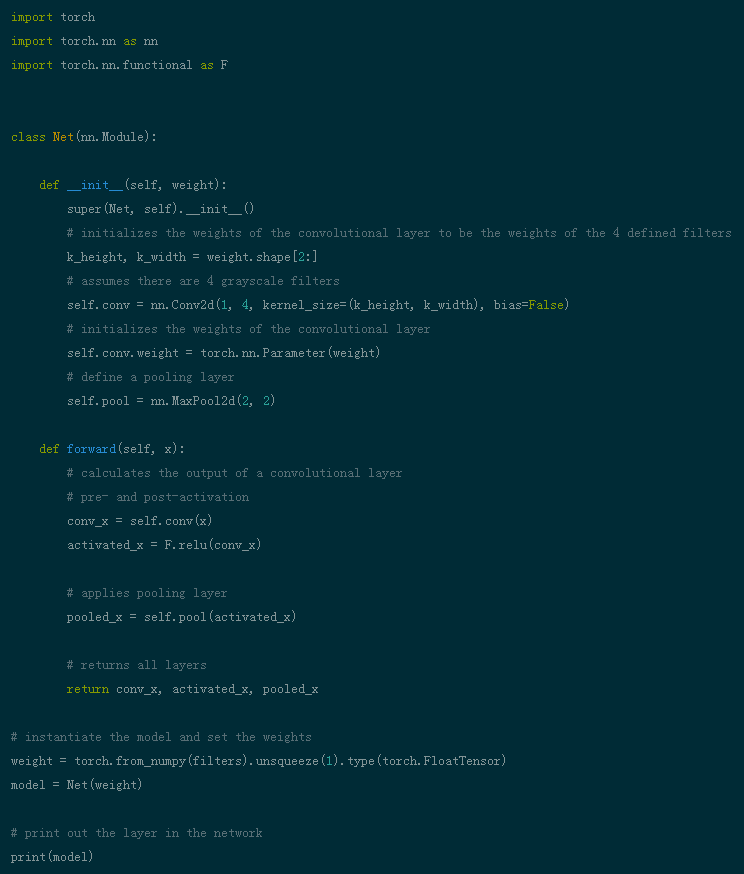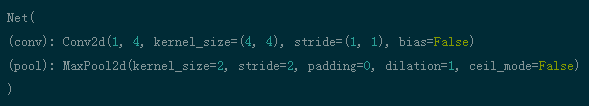def viz_layer(layer, n_filters= 4):    fig = plt.figure(figsize=(20, 20))            for i in range(n_filters):        ax = fig.add_subplot(1, n_filters, i+1)        ax.imshow(np.squeeze(layer[0,i].data.numpy()), cmap= gray )        ax.set_title( Output %s  % str(i+1))fig = plt.figure(figsize=(12, 6))fig.subplots_adjust(left=0, right=1.5, bottom=0.8, top=1, hspace=0.05, wspace=0.05)for i in range(4):    ax = fig.add_subplot(1, 4, i+1, xticks=[], yticks=[])    ax.imshow(filters[i], cmap= gray )    ax.set_title( Filter %s  % str(i+1))    gray_img_tensor = torch.from_numpy(gray_img).unsqueeze(0).unsqueeze(1)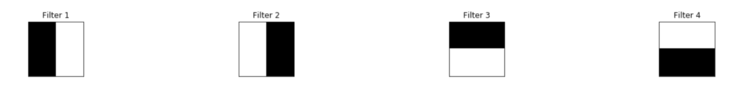viz_layer(activated_layer)viz_layer(pooled_layer)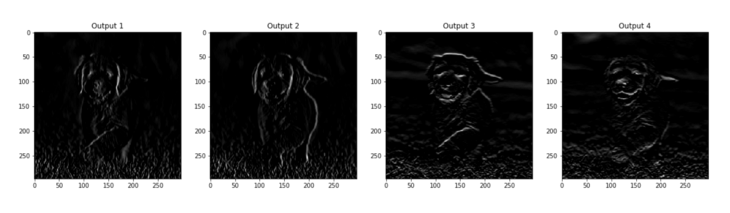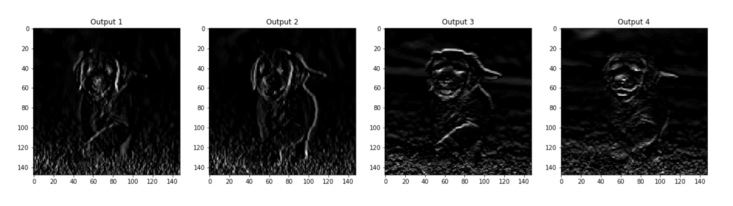via https://medium.com/better-programming/three-ways-to-use-the-walrus-operator-in-python-d5550f3a7dd0
0 收藏

### 作者的其它热门文章0 评论
0 收藏
0